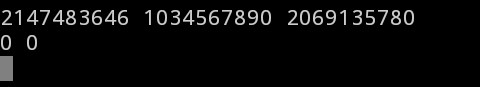# Highschool Mathematics

We do Algebra, Geometry, Matrix, Trigonometry, Calculus, Vector, Permutation - Combination, Probabil…

If you need help solving this problem, mention your approach and ask specific questions. Please avoid sharing your code and asking the Community to figure out “what’s wrong”.

Where the code is wrong?

``````#include<stdio.h>

int main() {
int a, b, c, d, e;
scanf("%d %d %d", &a, &b, &c);
d = b + c;
b = a * b/d;
c = a * c/d;
printf("%d %d", b, c);
return 0;
}
``````

The code gives wrong in the 6th test case.

Check your code with big numbers like 231-1. Your code fails to give write answers when it gets INT_MAX as x (the summation of the numbers).

@mashfiqur404
Dear why I gettin wa in last case???

@Rubel47
I checked your code with this case. But your code’s output dissapointed me.Your method is quite wrong. (But somehow it passed previous test cases!)

``````#include<iostream>
using namespace std;
int main()
{
unsigned long long n,m,r,div,mul2,mul,sum;
cin>>n>>m>>r;
sum=m+r;
div=n/sum;
mul=div*m;
mul2=div*r;
cout<<mul<<" "<<mul2<<endl;
}
``````

this code gives wrong 7th test case.why??

You’ll get CompilationError on this code. But I saw your original submission. Did you checked your code with the previous comment’s input? Your code fails there. Now find a solution.

7th test case produce wrong ans. bt why?

``````#include <stdio.h>
int main(){
long long x, m, n, temp;
scanf("%lld%lld%lld",&x,&m,&n);
temp=x/(m+n);
m=m*temp;
n=n*temp;
printf("%lld %lld\n",m,n);
return 0;
}
``````

“Have you checked your code for this input??”

``````#include<iostream>
using namespace std;
int main()
{
int y,p,q,a,b,c,x;
cin>>a>>b>>c;
x=b+c;
y=a/x;
p=y*b;
q=y*c;
cout<<p<<" "<<q;
}
``````

The code gives wrong in the 7th test case.

Have you checked your code for this input?

1 Like

#include<bits/stdc++.h>
using namespace std;
int main(){
long long int x,m,n,mult;
cin>>x>>m>>n;
mult=x/(m+n);
cout<<mmult<<" "<<nmult<<endl;
return 0;
}

why the testcase 7 is wrong? I got the same ans as u.

Watch previous replies.

Why is it showing wrong answer on test case 7😧

#include<stdio.h>
int main()
{
int x,m,n;
scanf(“%d%d%d”,&x,&m,&n);
int fst,scnd;
fst=(x/(m+n))*m;
scnd=x-fst;
printf(“%d %d”,fst,scnd);
}